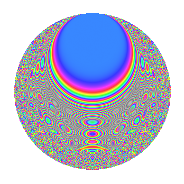# Properties

 Label 693.2.bnLevel 693 Weight 2 Character orbit bn Rep. character $$\chi_{693}(263,\cdot)$$ Character field $$\Q(\zeta_{6})$$ Dimension 184 Newform subspaces 1 Sturm bound 192 Trace bound 0

# Related objects

## Defining parameters

 Level: $$N$$ = $$693 = 3^{2} \cdot 7 \cdot 11$$ Weight: $$k$$ = $$2$$ Character orbit: $$[\chi]$$ = 693.bn (of order $$6$$ and degree $$2$$) Character conductor: $$\operatorname{cond}(\chi)$$ = $$693$$ Character field: $$\Q(\zeta_{6})$$ Newform subspaces: $$1$$ Sturm bound: $$192$$ Trace bound: $$0$$

## Dimensions

The following table gives the dimensions of various subspaces of $$M_{2}(693, [\chi])$$.

Total New Old
Modular forms 200 200 0
Cusp forms 184 184 0
Eisenstein series 16 16 0

## Trace form

 $$184q - 2q^{3} + 172q^{4} - 6q^{5} - 6q^{9} + O(q^{10})$$ $$184q - 2q^{3} + 172q^{4} - 6q^{5} - 6q^{9} + 3q^{11} - 30q^{12} - 6q^{14} - 6q^{15} + 148q^{16} - 24q^{20} - 6q^{22} + 12q^{23} + 78q^{25} - 36q^{26} - 14q^{27} - 4q^{31} + 5q^{33} - 56q^{36} - 4q^{37} - 54q^{42} - 39q^{44} - 46q^{45} - 98q^{48} - 2q^{49} - 24q^{53} - 10q^{55} - 60q^{56} + 6q^{58} - 6q^{60} + 88q^{64} - 60q^{66} - 4q^{67} - 10q^{69} - 42q^{70} + 16q^{75} - 58q^{78} - 108q^{80} + 26q^{81} + 18q^{86} - 3q^{88} - 30q^{89} - 36q^{91} + 78q^{92} + 12q^{93} - 4q^{97} + 70q^{99} + O(q^{100})$$

## Decomposition of $$S_{2}^{\mathrm{new}}(693, [\chi])$$ into newform subspaces

Label Dim. $$A$$ Field CM Traces $q$-expansion
$$a_2$$ $$a_3$$ $$a_5$$ $$a_7$$
693.2.bn.a $$184$$ $$5.534$$ None $$0$$ $$-2$$ $$-6$$ $$0$$

## Hecke Characteristic Polynomials

There are no characteristic polynomials of Hecke operators in the database